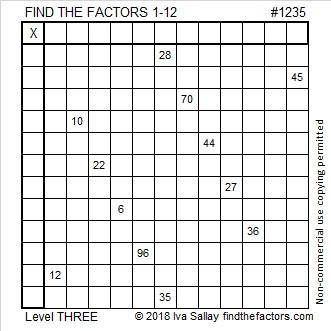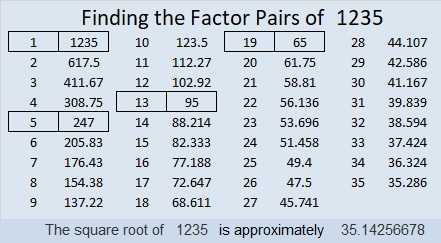# 1235 and Level 3

Do you know the greatest common factor of 28 and 35? If you do, then you can solve this puzzle by writing each number from 1 to 12 in both the first column and the top row. Since this is a level 3 puzzle, you can begin with the clues at the top of the puzzle and work your way down cell by cell. Good luck!Print the puzzles or type the solution in this excel file: 12 factors 1232-1241

Now I’ll share some facts about the number 1235:

• 1235 is a composite number.
• Prime factorization: 1235 = 5 × 13 × 19
• The exponents in the prime factorization are 1, 1, and 1. Adding one to each and multiplying we get (1 + 1)(1 + 1)(1 + 1) = 2 × 2 × 2 = 8. Therefore 1235 has exactly 8 factors.
• Factors of 1235: 1, 5, 13, 19, 65, 95, 247, 1235
• Factor pairs: 1235 = 1 × 1235, 5 × 247, 13 × 95, or 19 × 65
• 1235 has no square factors that allow its square root to be simplified. √1235 ≈ 35.142571235 is the hypotenuse of FOUR Pythagorean triples:
304-1197-1235 which is 19 times (16-63-65)
741-988-1235 which is (3-4-5) times 247
627-1064-1235 which is 19 times (33-56-65)
475-1140-1235 which is (5-12-13) times 95